## Example Questions

2 Next →

### Example Question #101 : Exponential Operations

Evaluate: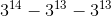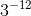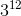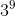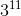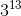Explanation:

When subtracting with exponents, we try to factor out some terms.

We can factor outto get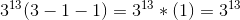.

### Example Question #111 : Exponents

Simplify: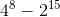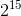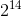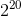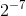Explanation:

Although we have different bases, we know that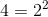.

Therefore,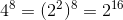.

Finally, we factor outto get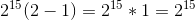.

### Example Question #12 : Exponents

If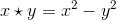, then what is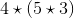?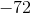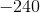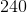Explanation:Follow the order of operations by solving the expression within the parentheses first.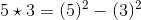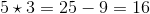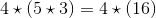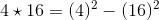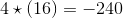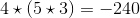2 Next →

### All SAT Math Resources